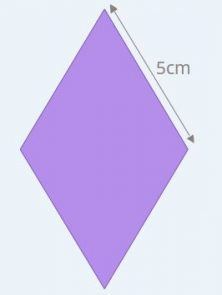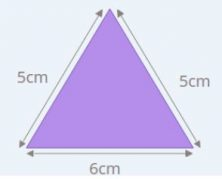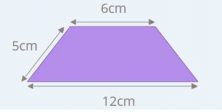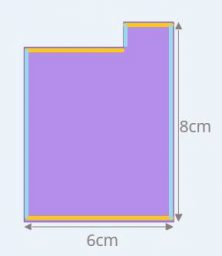Smartick is a fun way to learn math!Jan06

# How to Calculate a Perimeter: Part 2

In an earlier post, we learned how to calculate the perimeter of any polygon and formulas for regular polygons and rectangles. Today, we are going to learn how to calculate the perimeter of other types of polygons: rhombuses, isosceles triangles, isosceles trapezoids, and stepped polygons.

NOTE TO OUR READERS IN THE U.K. – This post uses the term trapezoid as it is used in the U.S.

### Calculating the perimeter of:

#### A rhombus

A rhombus has four equal sides. But not all of its angles are equal, only the angles that are opposite one another.Since the four sides are equal, we can multiply the length of one side by four in order to get the perimeter.

Perimeter = 4 x 5cm = 20cm

This rule is the same for squares because they also have four equal sides.

Perimeter of a rhombus  = 4 x the length of one side

#### An isosceles triangle

Isosceles triangles have two equal sides and one that is different.

To refresh your memory about the different types of triangles, take a look at this post.Given that two sides are equal and one is different, we just need to multiply the length of the repeated side by two and then add the length of the side that is different.

Perimeter = 5cm x 2 + 6cm = 16cm

So, for any isosceles triangle:

Perimeter of an isosceles triangle = length of repeated side x 2 + length of the side that is different

#### An isosceles trapezoid

The isosceles trapezoids have a special way of calculating their perimeter. They have two opposite sides that are equal and the other two sides, known as the bases (top and bottom), are parallel but different lengths.In this case, we need to multiply the length of one of the opposite sides by two and add the lengths of the two bases.

Perimeter = 5cm x 2 + 12cm + 6cm = 28cm

To calculate the perimeter for any isosceles trapezoid:

Perimeter of an isosceles trapezoid = length of opposite side x 2 + length of top base + length of bottom base

#### A stepped polygon

Stepped polygons have a very peculiar characteristic. The sum of the lengths of the sides that are parallel to the base measure the same as the length of the base. And the same goes for the sum of the lengths of the sides parallel to the height, which measure the same length as the height.So, to calculate the perimeter of any stepped polygon we can use the same formula that we use for rectangles because we can approach the sum of the lengths of the horizontal and vertical sides as if they were equal to the length of the base and height. It is as if the lengths of the base and height were repeated.

Perimeter = 2x (6cm + 8cm) = 28cm

The rule works for any stepped polygon of this type:

Perimeter of a stepped polygon = 2 x (base + height)